# Random Neuron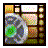## Watch the video tutorial on this subject!

### Random Neuron Functionality

Sometimes a little randomness is a good thing. When an ant is out searching for food and it is not picking up any scents on its antenna then it will start a random search to try and find food. Bacteria and other microorganisms do the same kind of thing. Random behavior comes into play quite often when an organism is searching for something or has exhausted its repertoire of normal options for dealing with a situation. The random neuron was created to handle the modeling of such behaviors. The random neuron uses three random variable equations to set its intrinsic current. These three equations control the amplitude of the intrinsic current that is generated, the duration between bursts, and the duration of bursts. A random number is generated between 0 and 100 and it is fed into these equations to find the final values that are used. This allows the user to configure the system using the gain functions to determine these time periods. So it is possible to setup a neuron that randomly fires an intrinsic current generated from a bell curve, with a burst duration generated by a linear function, and an inter-burst duration that is constant.

One question that might pop to mind is "why make things this complex?" Why not simply have a random number generator set the value of the intrinsic current at each time step? One big reason is that a random neuron that operated in that way would jump all over the place from time step to time step. On cycle 0 it could be at 10 na, cycle 1 it could jump to -5 na, and then on the next cycle jump up to 15 na. But it would not stay in any one place long enough for that setting to propagate through to the rest of the system. It would almost be like a white noise generator which would ultimately have no meaning. The second important reason is that this would limit the system to using only uniform random variables. But uniform random variables are not always the best system to solve a problem. Say, for instance, that the random neuron was going to be used to control the turning of an insect for random searching. Normally, the insect would only want to make small course changes. It would only want to make a 120o turn very rarely. It would want the ability to make a large turn like that, but not often. This type of behavior can be simulated very nicely with an inverted bell curve. The large bulk of the random numbers of the curve would then generate a modest, but increasing, intrinsic current. Numbers on the edges of the curve would generate large intrinsic currents. Also, using random variable equations for the burst and the inter-burst durations insures that a value of the intrinsic current is on for long enough that it can have an affect on the rest of the system. Using random variable equations gives the user flexibility in how they want to set those parameters.

### Random Firing Rate Neuron Properties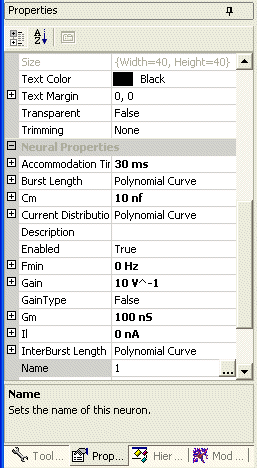Figure 1. The nodes available for the fast neural net plug-in module.

The random firing rate neuron model has all of the properties associated with a regular neuron: Cm, Gm, Vth, Fmin, and Gain. For a description of them please see the text that discusses the normal neuron. The properties that are unique for the pacemaker neuron are listed below with a description of each.

Burst Length
This is a gain function that determines the length of a random burst. A random number between 0 and 100 is chosen and fed into this gain function. The calculated value is the amount of time that the calculated burst current will be applied to this neuron. When this time span is over a new interburst-burst period will occur. See the gain function for details on how to configure gains.

Current Distribution
This is a gain function that determines the amplitude of the intrinsic current that will be applied during a burst. A random number between 0 and 100 is chosen and fed into this gain function. See the gain function for details on how to configure gains.

Il
The hyperpolarizing current that brings the membrane potential back down after it has been firing.
Default value: 0 na.
Acceptable range:Any Value.

Interburst Length
This is a gain function that determines the length between bursts. A random number between 0 and 100 is chosen and fed into this gain function. The calculated value is the amount of time that the Il current will be applied to this neuron. When this time span is over a new burst will occur. See the gain function for details on how to configure gains.

### Generating Random Intrinsic Currents

So how are the random values actually generated? There are two modes for the intrinsic current. It is either in a burst or not in a burst. It swaps back and forth between these two modes. If it is not in a burst and the timer runs out for that mode it switches to bursting. It first uses the current distribution gain function to determine the amplitude of the new burst. A number between 0 and 100 is randomly chosen. That number is fed into the gain function and the output is the current value that is used for the duration of this burst. If you want to always have the same burst amplitude then you simply need to use a uniform random variable. In other words, use a polynomial gain function and set all the values to zero except the intercept. Set it to the value of your burst current amplitude. Once the current is chosen it does the same thing to calculate the duration of the burst. It picks a random number between 0 and 100 and feeds that into the burst length gain function to find a time value for this burst. The intrinsic current stays at the calculated amplitude for the calculated amount of time. When it reaches the end of that time it determines the interburst duration by entering a random number between 0 and 100 into the interburst length gain function. The current that is used in the interburst is determined by the paramater Il. This gives you a great deal of freedom to configure the type of random bursting that will suite your needs.

### Random Neuron Output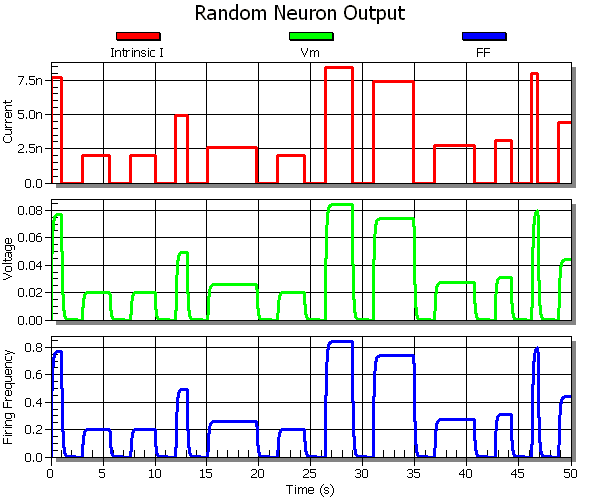Figure 2. The output from a random neuron that has a constant interburst duration.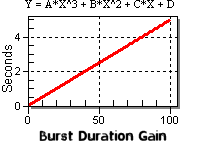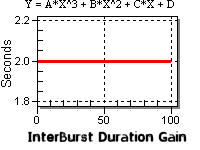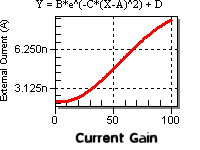Figure 3. The gain functions used to generate figure 2.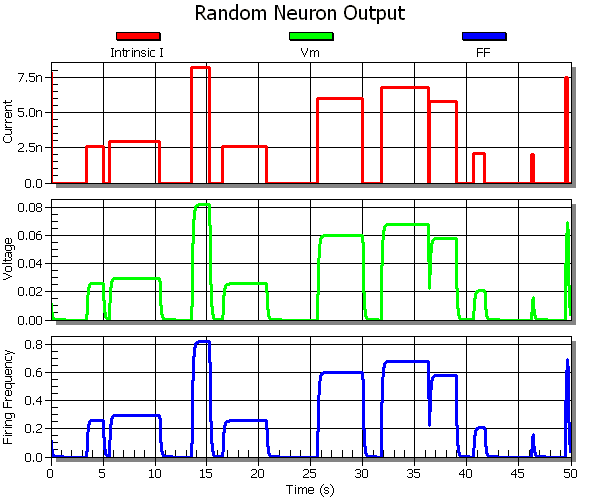Figure 4. The output from a random neuron that has a random interburst duration.

Figure 2 shows the firing pattern of a random neuron. The interburst duration was set to a constant value of 2 seconds That is why the time between bursts is so consistent. The gain functions used for that example are shown below it in figure 3. A straight line was used for the burst duration gain function. So as the random value varies between 0 and 100 the burst duration varies linearly between 0 and 5 seconds. The current gain used an inverted bell function that is shifted over slightly. The purpose for this is to try and keep low random values around the same current. Then the current increases almost linearly for the rest of the range. This biases the currents that are generated so that more low values come up because more of the random numbers produce low values. You could also do this with a sigmoidal graph.

For figure 4 the interburst duration gain was changed to be identical to the burst duration gain. This caused much more variability in the time between the bursts. Sometimes you get a new burst right on the heels of an ending burst, or it may take considerable time to get a new burst.

### Random Neuron Overview

Random neurons inject an element of chance into the system. The user can control these systems by setting the properties which control the size of the intrinsic currents and the durations of the burst and inter-burst times. Random neurons will not be used very often. But they do provide a very important function.